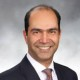## It’s Probable: You Have a Chance

As HR professionals wrap their heads around predictive human capital analytics, one of the capabilities required will be a firm grasp of probability. Not just relevant probability theory, but a feel for the numbers as well.

Why is this important? The outputs of predictive human capital models are typically probabilities, likelihoods and odds. If we are going to use predictive models, we need to wrap our heads around these special numbers.  This post deals with probabilities. A probability is simply a way of expressing knowledge or a belief that an event will occur or has occurred.  Subsequent posts will cover likelihoods and odds to complete the discussion.

In most cases, we will have an inkling or some sort of gut feel for what the prediction of a predictive human capital analytical model will be, based on training and experience. For example, if we are trying to model the probability of a new hire’s success in the company, based on his or her attributes, we typically have a feel for what sorts of candidates succeed in different business units, based on observations over the years.  Our predictive model will quantify this probability so that we can judge the impact of various factors.

Unless we are familiar with the mathematics underpinning the model, however, we might be surprised by the output. Unexpected results should always be examined to unearth the source of discrepancy from our expectations.  Sometimes the problem is the model; sometimes it is our expectations which are awry.

Two examples have emerged in the popular literature to illustrate how we cannot always trust our initial instincts with regard to probabilities.The Birthday Problem

The first example is known as “the birthday problem.” It has been around for a long time, but I was first confronted with it in an applied mathematics course at graduate school. It was one of the problems in the problem set assigned at the very first class. Fortunately, the solution was discussed by the professor in the next class (and fortunately for current students, is now widely available on the internet).

The problem is to figure out what is the probability that two people in a group of random individuals have the same birthday (day and month). Obviously, the larger the group, the larger is the probability.

Most people underestimate the likelihood that two people will have the same birthday. We have a prior notion that birthdays are rare (you have to wait 364 long days until your next one) and we only seldom encounter people with the same birthday as ours.

If you do the math right (there are a number of ways to arrive at the result), you get some fairly counter-intuitive results. With 20 people in a room, the probability of a shared birthday is as high as 41%. Increase the number to just 23 and you have even odds of a shared birthday (50% probability).

Looking at it another way, you only need 57 people to reach a 99% probability of a shared birthday. The table below summarizes the relationship.Try it out at your next meeting. If you don’t get a shared birthday, remember this was a probability – you are not guaranteed the result. Even with 366 people in the room, if one person has a birthday on February 29, all bets are off.

The Drug Test ProblemThe second example is typically framed as a drug test situation. Imagine a drug test that is known to correctly identify a drug user as testing positive 99% of the time and correctly identify a non-user as testing negative 99% of the time. That’s quite accurate by any measure. Now let’s assume that 0.5% of employees are drug users.

When the math is done right (using Bayes’ Theorem), the probability that someone who tests positive for the drug is actually a drug user is – hold your breath – 33%!  It’s more likely that the person is not a drug user!! Surely this can’t be – what’s going on here?

Despite the apparent sensitivity of the test, the low rate of drug use results in low accuracy. Basically, there’s a greater chance of false positives when the use rate is so low.

Both the birthday example and the drug test example are covered in greater detail in Wikipedia, including all the underlying math.

What are the conclusions? Here are two.

First, you need to have some understanding of basic probability theory. Being able to compute an expected value is essential. Knowing the difference between a probability and a conditional probability is important. One easy example is estimating turnover. Most people would measure turnover in a period as a percentage (say 17% if 17 people out of 100 left the organization). They would perhaps estimate that the probability of turnover is 17%. Fair enough. However to get an accurate estimate, one needs to look at individuals’ tenure and compute the probability of turnover after x years. This probability needs to be conditioned on the fact that they have not terminated for x years. I’ll cover this “life expectancy” notion of turnover in a subsequent post.

Second, gut feels for probabilities only work sometimes. It would be advisable to do the math or compute the model and come up with the actual probability. Of course, if the number does not agree with your hunch, you need to be able to get under the hood and work out the probabilities formally in order to convince yourself which number is right. With multiple variables and complicated or “advanced” predictive models, it is going to be very difficult to do the math yourself. At some point you have to trust the model. Which only means that you need to understand how he model works; it’s not enough to press a button, get the output and stick it onto a Powerpoint chart. Odds are that someone will call you on the probability underlying the prediction.